# Excel LOOKUP function with formula examples

The tutorial explains the vector and array forms of the Excel LOOKUP function and demonstrates typical and non-trivial uses of LOOKUP in Excel with formula examples.

One of the most frequent questions that every Excel user asks once in a while is this: "How do I look up a value on one sheet and pull a matching value to another sheet?". Of course, there can be many variations of the basic scenario: you may be looking for the closest match rather than exact match, you may want to search vertically in a column or horizontally in a row, evaluate one or multiple criteria, etc. However, the essence is the same - you need to know how to look up in Excel.

Microsoft Excel provides a handful of different ways to do lookup. To begin with, let's learn a function that is designed to handle the simplest cases of vertical and horizontal lookup. As you can easily guess, I am talking about the LOOKUP function.

## Excel LOOKUP function - syntax and uses

At the most basic level, the LOOKUP function in Excel searches a value in one column or row and returns a matching value from the same position in another column or row.

There are two forms of LOOKUP in Excel: Vector and Array. Each form is explained individually below.

## Excel LOOKUP function - vector form

In this context, a vector refers to a one-column or one-row range. Consequently, you use the vector form of LOOKUP to search one row or one column of data for a specified value, and pull a value from the same position in another row or column.

The syntax of the vector Lookup is as follows:

LOOKUP(lookup_value, lookup_vector, [result_vector])

Where:

• Lookup_value (required) - a value to search for. It can be a number, text, logical value of TRUE or FALSE, or a reference to a cell containing the lookup value.
• Lookup_vector (required) - one-row or one-column range to be searched. It must be sorted in ascending order.
• Result_vector (optional) - one-row or one-column range from which you want to return the result - a value in the same position as the lookup value. Result_vector must be the same size as lookup_range. If omitted, the result is returned from lookup_vector.

The following examples demonstrate two simple Lookup formulas in action.

#### Vertical Lookup formula - search in one-column range

Let's say, you have a list of sellers in column D (D2:D5) and the products they sold in column E (E2:E5). You are creating a dashboard where your users will enter the seller's name in B2 and you need a formula that would pull a corresponding product in B3. The task can be easily accomplished with this formula:

`=LOOKUP(B2,D2:D5,E2:E5)`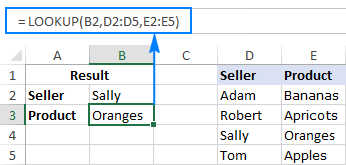To better understand the arguments, please have a look at this screenshot: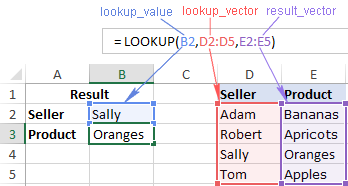#### Horizontal Lookup formula - search in one-row range

If your source data has a horizontal layout, i.e. the entries reside in rows rather than columns, then supply a one-row range in the lookup_vector and result_vector arguments, like this:

`=LOOKUP(B2,E1:H1,E2:H2)`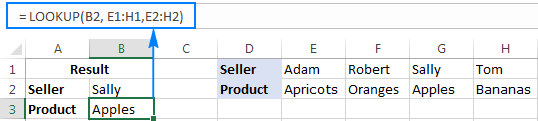In the second part of this tutorial, you will find a few more Excel Lookup examples that solve more complex tasks. In the meantime, please remember the following simple facts that will help you bypass possible pitfalls and prevent common errors.

### 5 things you should know about vector form of Excel LOOKUP

1. Values in lookup_vector should be sorted in ascending order, i.e. from smallest to largest or from A to Z, otherwise your Excel Lookup formula may return an error or incorrect result. If you need to do lookup on unsorted data, then use either INDEX MATCH or OFFSET MATCH.
2. Lookup_vector and result_vector must be a one-row or one-column range of the same size.
3. The LOOKUP function in Excel is case-insensitive, it does not differentiate uppercase and lowercase text.
4. Excel LOOKUP works based on approximate match. More precisely, a Lookup formula searches for exact match first. If it cannot find the lookup value exactly, it looks up the next smallest value, i.e. the largest value in lookup_vector that is less than or equal to lookup_value.

For example, if your lookup value is "5", the formula will search it first. If "5" is not found, it will search "4". If "4" is not found, it will search "3", and so on.

5. If lookup_value is smaller than the smallest value in lookup_vector, Excel LOOKUP returns the #N/A error.

## Excel LOOKUP function - array form

The array form of the LOOKUP function searches the specified value in the first column or row of the array and retrieves a value from the same position in the last column or row of the array.

The array Lookup has 2 arguments, both of which are required:

LOOKUP(lookup_value, array)

Where:

• Lookup_value - a value to search for in an array.
• Array - a range of cells where you want to search for the lookup value. The values in the first column or row of the array (depending on whether you do V-lookup or H-lookup) must be sorted in ascending order. Uppercase and lowercase characters are deemed equivalent.

For example, with the seller names located in the first column of the array (column A) and order dates in the last column of the array (column C), you can use the following formula to search the name and pull the matching date:

`=LOOKUP(B2,D2:F5)`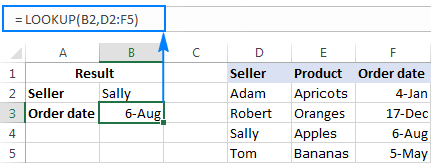Note. The array form of the Excel LOOKUP function should not be confused with Excel array formulas. Although it operates on arrays, LOOKUP is still a regular formula, which is completed in the usual way by pressing the Enter key.

### 4 things you should know about array form of Excel LOOKUP

1. If array has more rows than columns or the same number of columns and rows, a Lookup formula searches in the first column (horizontal lookup).
2. If array has more columns than rows, Excel LOOKUP searches in the first row (vertical lookup).
3. If a formula can't find the lookup value, it uses the largest value in the array that is less than or equal to lookup_value.
4. If the lookup value is smaller than the smallest value in the first column or row of the array (depending on the array dimensions), a Lookup formula returns the #N/A error.

Important note! The functionality of the Excel LOOKUP array form is limited and we don't recommend using it. Instead, you can use the VLOOKUP or HLOOKUP function, which are the improved versions to do vertical and horizontal lookup, respectively.

## How to use LOOKUP function in Excel - formula examples

Although there exist more powerful functions to look up and match in Excel (which is the subject of our next tutorial), LOOKUP comes in handy in many situations, and the following examples demonstrate a couple of non-trivial uses. Please note, all of the below formulas use the vector form of Excel LOOKUP.

### Look up a value in the last non-blank cell in a column

If you have a column of dynamically populated data, you may want to pick the most recently added entry, i.e. get the last non-empty cell in a column. For this, use this generic formula:

LOOKUP(2, 1/(column<>""), column)

In the above formula, all arguments except for the column reference are constants. So, to retrieve the last value in a specific column, you just need to supply the corresponding column reference. For example, to extract the value of the last non-blank cell in column A, use this formula:

`=LOOKUP(2, 1/(A:A<>""), A:A)`

To get the last value from other columns, modify the column references like shown in the screenshot below - first reference is the column to be checked for blank/non-blank cells, and the second reference is the column to return the value from: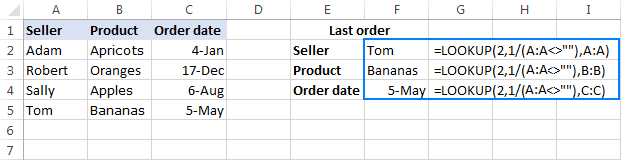#### How this formula works

In the lookup_value argument, you supply 2 or any other number greater than 1 (in a moment, you will understand why).

In the lookup_vector argument, you put this expression: 1/(A:A<>"")

• First, you perform the logical operation A:A<>"" that compares each cell in column A with an empty string and returns TRUE for empty cells and FALSE for non-empty cells. In the above example, the formula in F2 returns this array: {TRUE;TRUE;TRUE;TRUE;FALSE...}
• Then, you divide the number 1 by each element of the above array. With TRUE equating to 1 and FALSE equating to 0, you get a new array consisting of 1's and #DIV/0! errors (the result of dividing by 0), and this array is used as lookup_vector. In this example, it's {1;1;1;1;#DIV/0!...}

Now, how it comes that the formula returns the last non-empty value in a column, given that lookup_value does not match any element of lookup_vector? The key to understanding the logic is that Excel LOOKUP searches with approximate match, i.e. when the exact lookup value is not found, it matches the next biggest value in lookup_vector that is smaller than lookup_value. In our case, lookup_value is 2 and the largest value in lookup_vector is 1, so LOOKUP matches the last 1 in the array, which is the last non-empty cell!

In the result_vector argument, you reference the column from which you want to return a value, and your Lookup formula will fetch the value in the same position as the lookup value.

Tip. If you wish to get the number of the row holding the last value, then use the ROW function to retrieve it. For example: `=LOOKUP(2,1/(A:A<>""),ROW(A:A))`

### Look up a value in the last non-blank cell in a row

If your source data is laid out in rows rather than columns, you can get the value of the last non-empty cell using this formula:

LOOKUP(2, 1/(row<>""), row)

In fact, this formula is nothing else but a slight modification of the previous formula, with the only difference that you use the row reference instead of the column reference.

For example, to get the value of the last non-empty cell in row 1, use this formula:

`=LOOKUP(2, 1/(1:1<>""), 1:1)`

The following screenshot shows the result: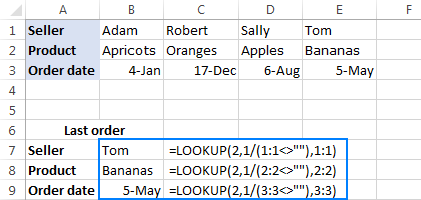### Get a value associated with the last entry in a row

With just a little creativity, the above formula can easily be customized for solving other similar tasks. For instance, it can be used to get a value associated with the last instance of a specific value in a row. This may sound a bit obscure, but the following example will make things easier to understand.

Assuming you have a summary table where column A contains the seller names and subsequent columns contain data of some kind for each month. In this example, a cell contain "yes" if a given seller has closed at least one deal in a given month. Our goal is to get a month associated with the last "yes" entry in a row.

The task can be solved by using the following LOOKUP formula:

`=LOOKUP(2, 1/(B2:H2="yes"), \$B\$1:\$H\$1)`

The formula's logic is basically the same as described in the first example. The difference is that you use the "equal to" operator ("=") instead of "not equal to" ("<>") and operate on rows instead of columns.

The following screenshot demonstrates a result: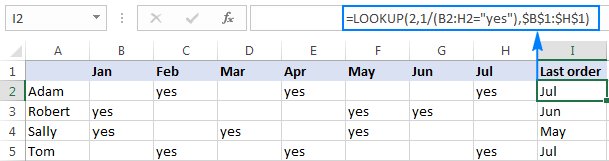### Lookup as alternative to nested IFs

In all of the Lookup formulas we've discussed so far, the lookup_vector and result_vector arguments were represented by range references. However, the syntax of the Excel LOOKUP function allows supplying the vectors in the form of a vertical array constant, which enables you to replicate the functionality of nested IF with a more compact and easy-to-read formula.

Let's say, you have a list of abbreviations in column A and you want to replace them with full names, where "C" stands for "Completed", "D" is "Development, and "T" is "Testing". The task can be accomplished with the following nested IF function:

`=IF(A2="c", "Completed", IF(A2="d", "Development", IF(A2="t", "Testing", "")))`

Or, by using this Lookup formula:

`=LOOKUP(A2, {"c";"d";"t"}, {"Completed";"Development";"Testing"})`

As shown in the screenshot below, both formulas yield identical results: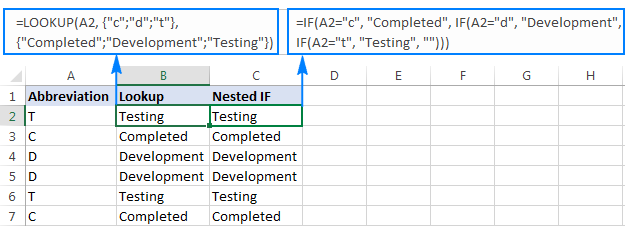Note. For an Excel Lookup formula to work correctly, the values in lookup_array should be sorted from A to Z or from smallest to largest.

If you are pulling values from a lookup table, then you can embed a Vlookup function in the lookup_value argument to retrieve a match.

Assuming the lookup value is in cell E2, the lookup table is A2:C7, and the column of interest ("Status") is the 3rd column in the lookup table, the following formula does the job:

`=LOOKUP(VLOOKUP(E2, \$A\$2:\$C\$7, 3, FALSE), {"c";"d";"t"}, {"Completed";"Development";"Testing"})`

As demonstrated in the screenshot below, the formula retrieves the project status from the lookup table and replaces an abbreviation with the corresponding word: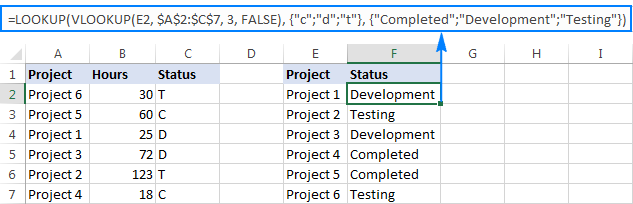Tip. If you are using Excel 2016 as part of an Office 365 subscription, you can use the SWITCH function for similar purposes.

I hope these examples have shed some light on how the LOOKUP function works. To better understand the formulas, you are welcome to download these Excel Lookup examples. In the next tutorial, we will discuss a few other ways to do lookup in Excel and explain which Lookup formula is best to be used in which situation. I thank you for reading and hope to see you on our blog next week!

## You may also be interested in

1. I am trying to use the LOOKUP function, I have codes for some persons, these codes are combination of numerical and alphabetical characters. the LOOKUP function is working very well except when the code is starting with 0 or 1. Are there any explanation for that, and what can I do.
Thank you

• I’m sorry, but your task is not entirely clear to me. For me to be able to help you better, please describe your task in more detail. Please specify what formula you used and what problem or error occurred. Give an example of the source data and the expected result.
It’ll help me understand it better and find a solution for you.

• Thank you for your response, I prepared an illustration to clarify my point
In this example, I have a unique code for each person, some codes have been selected by other department, and I have to send these persons emails. So I used the lookup function to check who of my list has to receive an email. There is an example of the lookup function that I used.
I checked by the code in cell A2 (Lookup_value), in the vector cell A16 to cell A19 (lookup_vector).
As I understand if the contents of A2 is included in the lookup vector, this value will be return.
Using the shown formula, all answers were correct (either True or False) except the one with code "07E636D0"

Code Name Send Email?
56E4FE26 John FALSE "=LOOKUP(\$A2,\$A\$16:\$A\$19)=\$A2"
ED58035C Mark FALSE
E58C9E88 Sarah TRUE
16DC22EA George TRUE
3BBDD787 Usama TRUE
6725593E Jasmin FALSE
07E636D0 Michel #N/A
35F11E43 Hasmid FALSE
A23A7058 Ernieza FALSE
90BFA9D0 Akmal FALSE

Code of Selected Persons
16DC22EA
3BBDD787
E58C9E88
07E636D0

• Hi! The values in the lookup_vector (A16 to A19) must be sorted in ascending order, i.e. from smallest to largest or A to Z, or otherwise your lookup formula may return an error or incorrect result. Read the article above carefully.

• Thank you, I tried to sort the lookup_vector, as in the following example, but it gave me same error

Code Name Send Email?
07E636D0 Aref #N/A "=LOOKUP(\$A2,\$A\$20:\$A\$31)=\$A2"
13C6D63C Luay #N/A
16DC22EA Saher #N/A
24B05219 Mahm #N/A
24B0EB0F Irene #N/A
250C4E31 Ihab #N/A
2749E54B Moham #N/A
2A4B7669 Hnay #N/A
35F11E43 Sarah #N/A
3BBDD787 Cani #N/A
48441A3A Ibraham FALSE
56E4FE26 Hana FALSE
5D723A44 William C FALSE

Code of Selected Persons
46DBF3C2
4CD83BD4
62CB0960
62CB0960
90BFA9D0
94F6970A
9EA377EA
AFA0A326
EE82CFBC
F4CE3F54
F4CE3F54

• It's hard to find values that aren't there. Take a closer look at your data.

2. I have unique student IDs in column, and in other columns I have their subjects. I want to generate exam fee slip for them. I have subject and its fee in other columns. for receipt/slip generation, the formula against the subject should read if there is fee mentioned in the desired column under the subject. It should automatically populate it in row on the slip page alongwith its respective fee in the next column. I would prefer match index formula since my columns in data page will may change. My data is in such format
C:C D:D
\$ 1000 \$1100
Accouting Economics
School Id #
columns
B;B
16500
16501
16502
the starting point I think is; if the desired cell is filled( non-empty), the next step

3. =LOOKUP(AE4, {"MBB";"PBB";"CIMB"}, {"CB";"PB";"MB"})

Appreciate could point out where I went wrong with the above formula. It worked with the following
MBB - MB
PBB - PB

but when input with CIMB it returns #N/A instead of CB

Thanks

• Hi!
Please note that Lookup_vector must be sorted in ascending order. You didn't do it.

4. I am trying to create a sheet for classes out company offers. I have the class name up to about 25 classes. When I put the class name in I want to check 2 other columns for an Value (X) to see if they are a member or non member. and put the price of the class in the next column. I have an array with all the class names and prices for both but i cant figure out how to do it.

• Hi! I'm sorry, I'm afraid these pieces of info are not enough to give you a formula. To find the value in the other two columns, try using this guide: How to do sequential VLOOKUPs in Excel.
If this does not help, explain the problem in detail.

5. I am looking to pull data from one tab into a consolidated table, The tab I am pulling from matches a customer column and date column and needs to pull from a column that may have multiple cells to give a total. What would be the best method to accomplish this in excel.

• Hi!
Unfortunately, without seeing your data it is difficult to give you any advice. If you have a specific question about the operation of a function or formula, I will try to answer it.

6. Hi Svetlana,

Good morning!

I have a duty roster of nurses for the month of Feb. Below here is a sample. I want a formula that can give me the last two days' duty. (E.g EN). Each month we have days ending. I have tried =CONCATENATE but it's not working every month.

E E N N O O M M E E N N O O M M E E N N N O O M M E E N

• Hi!
I’m sorry but your task is not entirely clear to me. Could you please describe it in more detail? What result do you want to get?